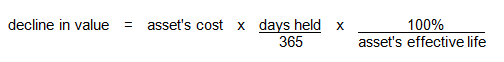• ## How to work out the decline in value

You decide whether to calculate the decline in value of a depreciating asset using either the:

In some cases, you must use the same method used by the former holder of the asset. For example if you acquire the asset from an associate such as your spouse or business partner. For some intangible depreciating assets, including an item of intellectual property, you must always use the prime cost method.

### Prime cost method

Under the prime cost method, the decline in value is generally calculated as a constant percentage of the asset's cost and reflects a uniform decline in value over time. The formula is:The cost of an asset includes both the amount you pay for it as well as any additional amounts you spend on transporting it and installing it in position. Cost also includes amounts you spend on improving the asset.

In some circumstances, such as when you change the effective life or cost of an asset, an adjusted prime cost formula must be used.

### Diminishing value method

Under the diminishing value method, the decline in value is calculated using the asset's base value. The base value of an asset is broadly its cost plus any costs incurred on the asset since you first held it (say on improvements) less the decline in value of the asset up to the end of the prior year.

The formula for the diminishing value method is:if you started to hold the asset prior to 10 May 2006, orif you started to hold the asset on or after 10 May 2006.

This method assumes the decline in value each year is a constant proportion of the amount not yet written-off and produces a progressively smaller decline in value over time.

Example 1

Depreciating Asset Pty Ltd acquires an asset for \$10,000 on 1 July 2013 and starts to use it wholly for taxable purposes from that day. The effective life of the asset is 10 years.

If Depreciating Asset Pty Ltd chose to use the diminishing value method to calculate the asset's decline in value, the company's deductions over the next two years would be:

• 2013–14 income year: 10,000 × (365/365) × (200%/10) = \$2,000
• 2014–15 income year: (10,000 − 2,000) × (365/365) × (200%/10) = \$1,600.

If the company chose to use the prime cost method to calculate the asset's decline in value, the deductions over the two years would be:

• 2013–14 income year: 10,000 × (365/365) × (100%/10) = \$1,000
• 2014–15 income year: 10,000 × (365/365) × (100%/10) = \$1,000.

Example 2

A deduction for the decline in value of a depreciating asset is reduced by the extent it is not used for a taxable purpose. For example, if an asset is used 40% of the time for a private purpose, the deduction for its decline in value is reduced by 40%.

The decline in value of certain assets costing \$300 or less will be the cost, that is, you will be entitled to an immediate deduction.

End of example